Courses

# Mathematics Test 5 - Definite And Indefinite Integration, Differential Equation

## 30 Questions MCQ Test JEE Main Mock Test Series 2020 & Previous Year Papers | Mathematics Test 5 - Definite And Indefinite Integration, Differential Equation

Description
This mock test of Mathematics Test 5 - Definite And Indefinite Integration, Differential Equation for JEE helps you for every JEE entrance exam. This contains 30 Multiple Choice Questions for JEE Mathematics Test 5 - Definite And Indefinite Integration, Differential Equation (mcq) to study with solutions a complete question bank. The solved questions answers in this Mathematics Test 5 - Definite And Indefinite Integration, Differential Equation quiz give you a good mix of easy questions and tough questions. JEE students definitely take this Mathematics Test 5 - Definite And Indefinite Integration, Differential Equation exercise for a better result in the exam. You can find other Mathematics Test 5 - Definite And Indefinite Integration, Differential Equation extra questions, long questions & short questions for JEE on EduRev as well by searching above.
QUESTION: 1

### The differential equation of all circles which pass through the origin and whose centres lie on y-axis is

Solution:
Toolbox:
Equation of a family of circles in (x−h)^2+(y−k)^2=a^2 where (h,k) are the centers and a is the radius.
If the given equation has 'n' arbitary constants, then the given equation will be of h order

We are asked to form the differential equations of all circles which pass through the orgin and whose centers lies on y-axis
Since it is given that the center lies on the y-axis, the sketch of the circle is as shown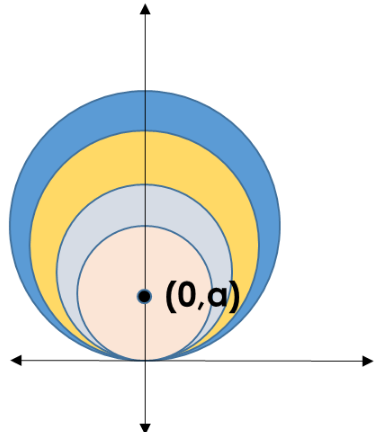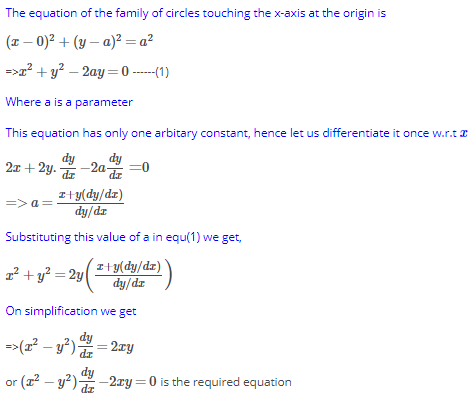QUESTION: 2

Solution:
QUESTION: 3

### Order and degree of differential equation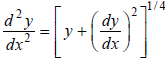are

Solution:
QUESTION: 4

Differential equation for y = A cos αx + B sin αx where A and B are arbitrary constants is

Solution:
QUESTION: 5
The solution of the differential equation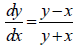is
Solution:
QUESTION: 6

The integrating factor of the different equation dy/dx ( x log x ) + y = 2 log x is given by:

Solution:
QUESTION: 7

Solution of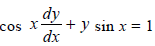is

Solution:
QUESTION: 8

The solution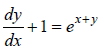is

Solution:
QUESTION: 9

Solution of differential equation xdy – ydx = 0 represents

Solution:
QUESTION: 10

Integration factor of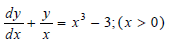is

Solution:
QUESTION: 11

A continuously differentiable function  y = f(x) ∈ (0,π ) satisfying  y = 1 + y, y (0) = 0 = y(π)is

Solution:
QUESTION: 12

The solution of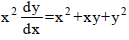is

Solution:
QUESTION: 13
A primitive of sin x cos x is
Solution:
QUESTION: 14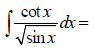Solution:
QUESTION: 15
If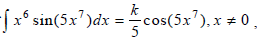then
Solution:
QUESTION: 16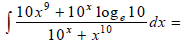Solution:
QUESTION: 17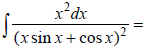Solution:
QUESTION: 18

The primitive of | x |, when x < 0 is

Solution:
QUESTION: 19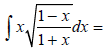Solution:
QUESTION: 20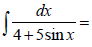Solution:
QUESTION: 21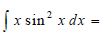Solution:
QUESTION: 22

The value of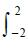( a x3 + bx + c) dx =  depends on

Solution:
QUESTION: 23Solution:
QUESTION: 24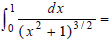Solution:
QUESTION: 25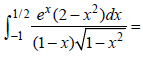Solution:
QUESTION: 26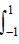Sin 11 x dx =

Solution:
QUESTION: 27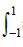Sin3 x cos2 x dx =

Solution:
QUESTION: 28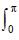Cos3 x dx =

Solution:
QUESTION: 29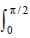| Sin π x | dx =

Solution:
QUESTION: 30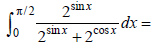Solution: